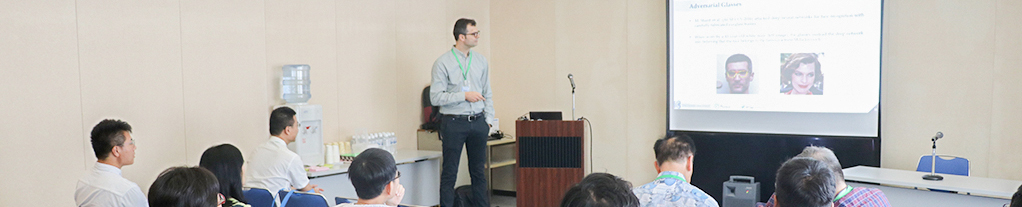To The Top!Topics of Submission
Topics in Wavelet Analysis
• Algorithms
• Approximation Theory
• Bases and Frames Theory
• Equation
• General Theory
• Hardware and Software Implementation of Wavelet Transforms
• Integral Transforms
• Spline Theory
• Time-Frequency Analysis
• Wavelet and Differential and Integral Equations
• Wavelet and Functional Analysis
• Wavelet and Numerical Analysis
• Wavelet and Sampling
• Wavelet and Statistics
• Wavelet Neural Network
• Wavelets and Filter Banks
Topics in Pattern Recognition & Related Fields
• Analysis and detection of singularities
• Animation image analysis
• Artificial Life
• Biomedical imaging
• Biometrics
• Classification
• Cluster analysis
• Deformation analysis
• Descriptor of shapes
• Diagnosis of faults
• Document analysis
• Emotion Computation
• Enhancement and restoration
• Feature extraction
• Hand Gestures Classification
• Handwritten and printed character recognition
• Human face recognition
• Image compression
• Image fusion
• Image indexing and retrieval
• Image recovery
• Intelligent Control System
• Intelligent Human Machine Interface
• Intelligent Robot
• Invariant representation of patterns
• Iris pattern recognition
• Learning Theory
• Machine Vision
• Medical Image Analysis
• Noise reduction
• Nonstationary stochastic processing
• Range imaging and detection
• Segmentation
• Seismological signal processing
• Signal estimation
• Stochastic pattern recognition
• Texture analysis and classification
• Visualization
• Watermarking